Topic 4 Consumer Behavior Utility Like elasticity Utility

• Slides: 42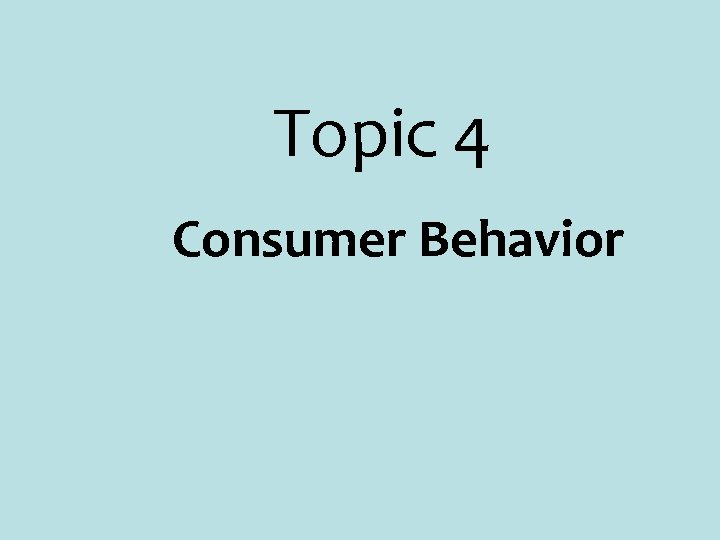Topic 4 Consumer BehaviorUtility § Like elasticity, Utility is another fancy name for satisfaction or happiness § Utility refers to satisfaction derived from consumption of goods and services. § To understand consumer behavior, we must know the difference between Total Utility and Marginal Utility 7 -2Total Utility Cumulative satisfaction derived from consumption of a specific quantity of goods or services. Example: § Lets look at Bob’s satisfaction schedule from ice cream consumption. 7 -3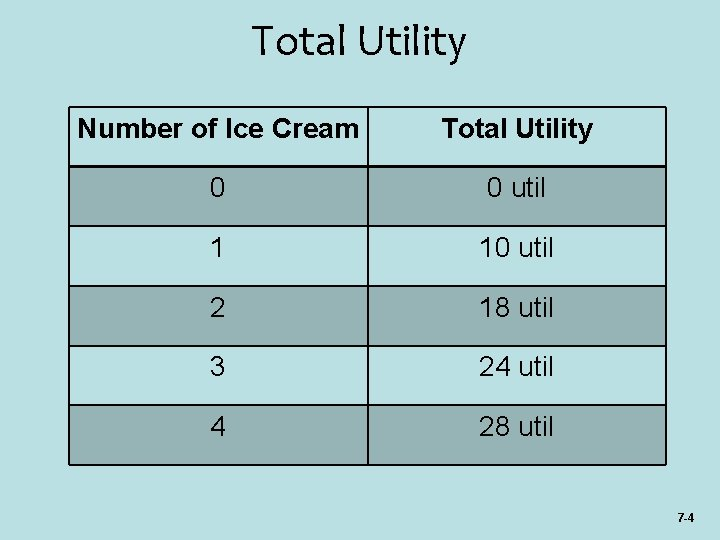Total Utility Number of Ice Cream Total Utility 0 0 util 1 10 util 2 18 util 3 24 util 4 28 util 7 -4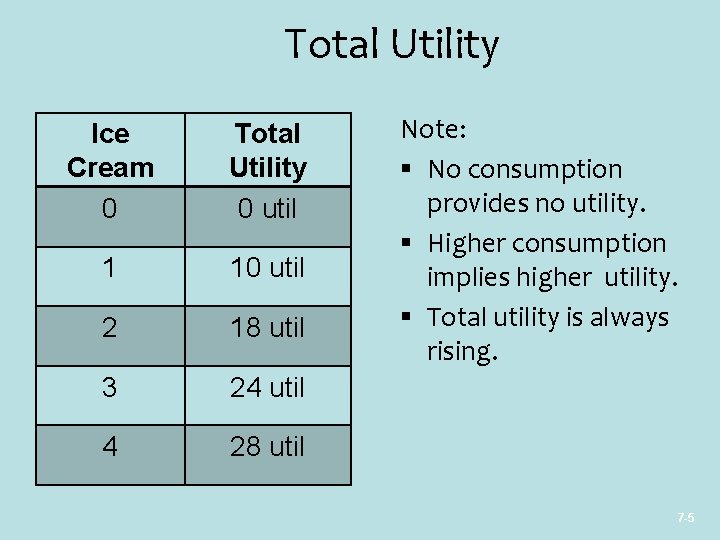Total Utility Ice Cream 0 Total Utility 0 util 1 10 util 2 18 util 3 24 util 4 28 util Note: § No consumption provides no utility. § Higher consumption implies higher utility. § Total utility is always rising. 7 -5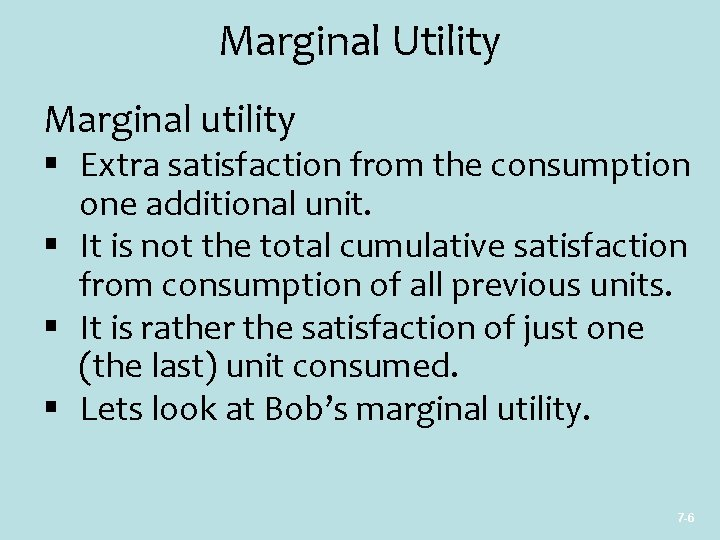Marginal Utility Marginal utility § Extra satisfaction from the consumption one additional unit. § It is not the total cumulative satisfaction from consumption of all previous units. § It is rather the satisfaction of just one (the last) unit consumed. § Lets look at Bob’s marginal utility. 7 -6Marginal Utility Number of Ice Cream 0 Total Utility 0 util Marginal Utility Undefined 1 10 util 2 18 util 3 24 util 6 util 4 28 util 4 util 7 -Marginal Utility Ice Cream 0 Marginal Utility Undefined 1 10 util 2 8 util 3 6 util 4 4 util Note: § Needs two units to compare marginal utility. § Marginal utility falls with consumption. § Can be even negative. § When MU is negative, TU must fall from previous TU 7 -8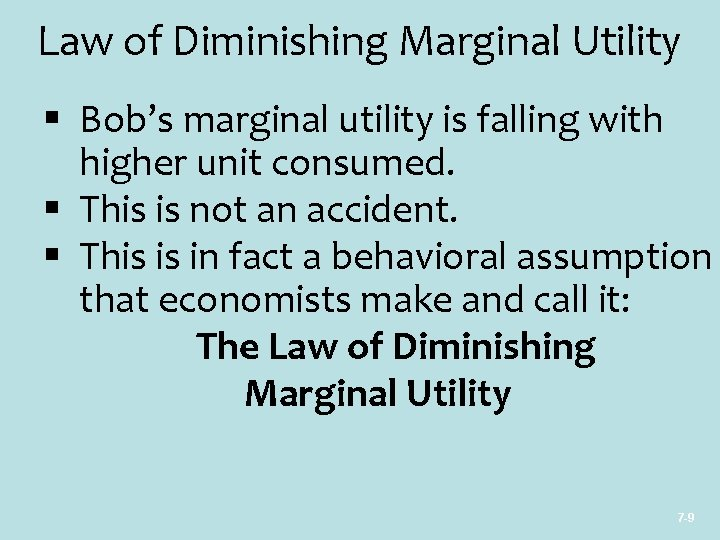Law of Diminishing Marginal Utility § Bob’s marginal utility is falling with higher unit consumed. § This is not an accident. § This is in fact a behavioral assumption that economists make and call it: The Law of Diminishing Marginal Utility 7 -9Utility Graphically TU (1) (2) (3) Tacos Total Marginal Consumed Utility, Per Meal Utils 0 1 2 3 4 5 6 7 0 ] 18 ] 24 ] 28 ] 30 ] 28 10 Total Utility 30 TU 20 10 8 6 0 4 MU 2 0 -2 1 2 3 4 5 6 Q 7 Marginal Utility 10 8 6 4 2 0 -2 MU 1 2 3 4 5 6 Units Consumed Per Meal 7 7 -10 QTheory of Consumer Behavior § This theory tells us how consumers maximize utility. § It tells us how much of each goods or services consumer should buy to maximize total utility. § It finds utility maximizing: § Quantities (or Q*) § When Income (or M) is fixed § When Prices (or P) are fixed 7 -11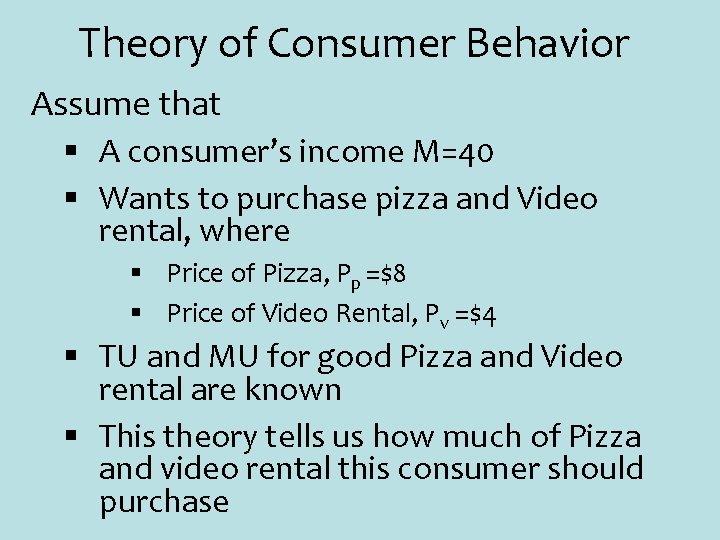Theory of Consumer Behavior Assume that § A consumer’s income M=40 § Wants to purchase pizza and Video rental, where § Price of Pizza, Pp =\$8 § Price of Video Rental, Pv =\$4 § TU and MU for good Pizza and Video rental are known § This theory tells us how much of Pizza and video rental this consumer should purchase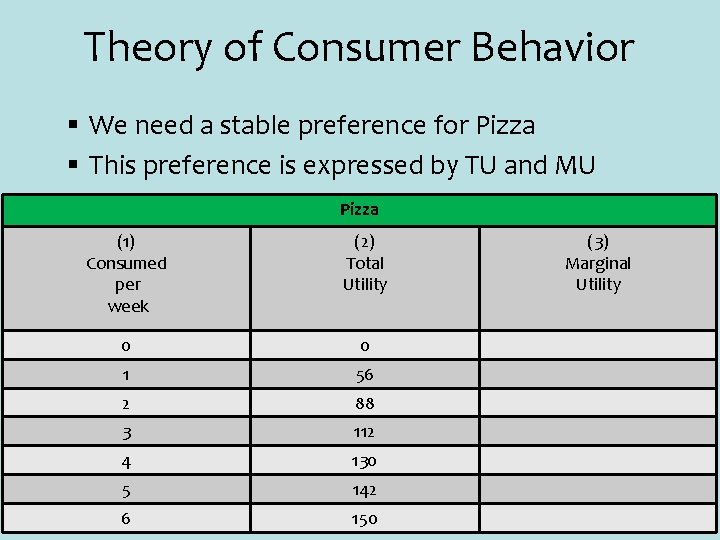Theory of Consumer Behavior § We need a stable preference for Pizza § This preference is expressed by TU and MU Pizza (1) Consumed per week (2) Total Utility 0 0 1 56 2 88 3 112 4 130 5 142 6 150 (3) Marginal Utility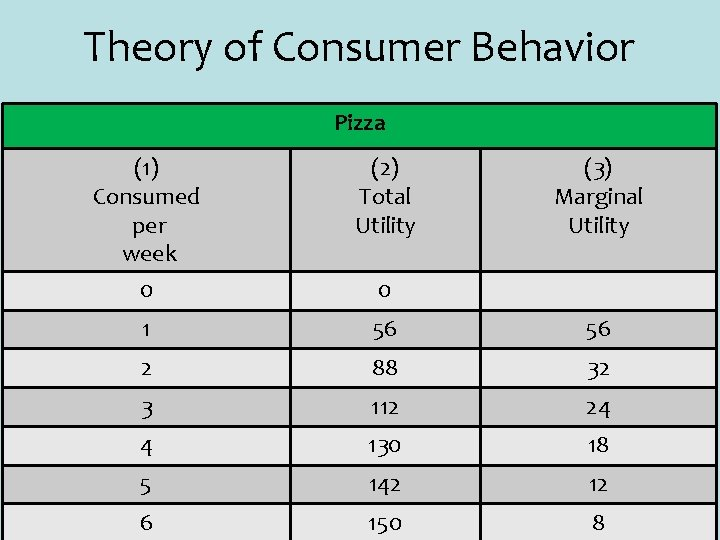Theory of Consumer Behavior Pizza (1) Consumed per week 0 (2) Total Utility (3) Marginal Utility 1 56 56 2 88 32 3 112 24 4 130 18 5 142 12 6 150 8 0Theory of Consumer Behavior § Similarly we need a stable Preference for Video Rental expressed by TU and MU Video Rental (1) Viewed per week (2) Total Utility 0 0 1 40 2 68 3 88 4 100 5 108 6 114 (3) Marginal Utility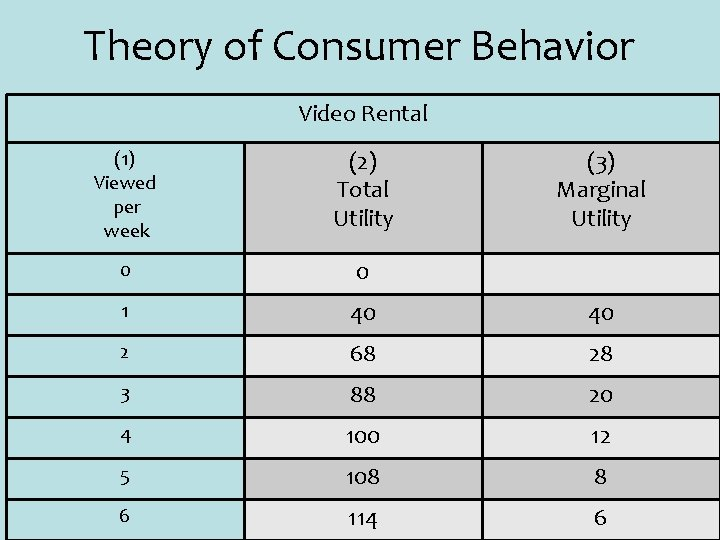Theory of Consumer Behavior Video Rental (1) Viewed per week (2) Total Utility (3) Marginal Utility 0 0 1 40 40 2 68 28 3 88 20 4 100 12 5 108 8 6 114 6Theory of Consumer Behavior § Which one would you consume first? § Is it your first pizza (with 56 MU)? Pizza (1) Consumed per week (2) Total Utility 0 0 1 56 2 Video Rental (3) Marginal Utility (1) Viewed per week (2) Total Utility (3) Marginal Utility 0 0 56 1 40 40 88 32 2 68 28 3 112 24 3 88 20 4 130 18 4 100 12 5 142 12 5 108 8 6 150 8 6 114 6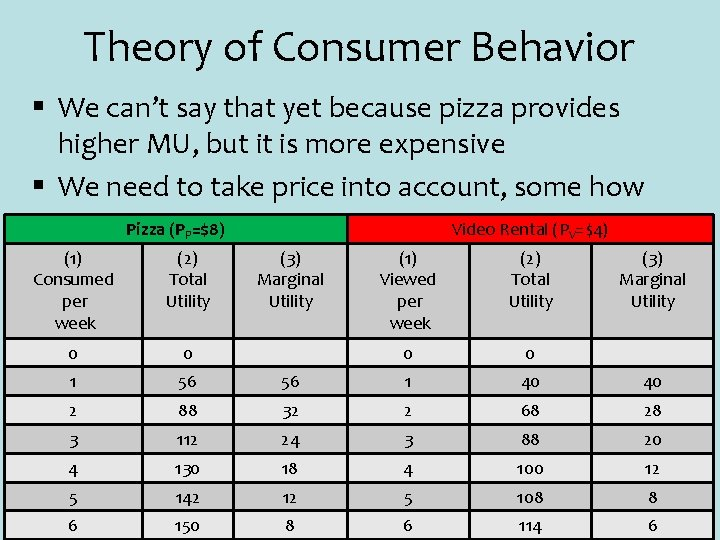Theory of Consumer Behavior § We can’t say that yet because pizza provides higher MU, but it is more expensive § We need to take price into account, some how Pizza (PP=\$8) (1) Consumed per week (2) Total Utility 0 0 1 56 2 Video Rental (PV=\$4) (3) Marginal Utility (1) Viewed per week (2) Total Utility (3) Marginal Utility 0 0 56 1 40 40 88 32 2 68 28 3 112 24 3 88 20 4 130 18 4 100 12 5 142 12 5 108 8 6 150 8 6 114 6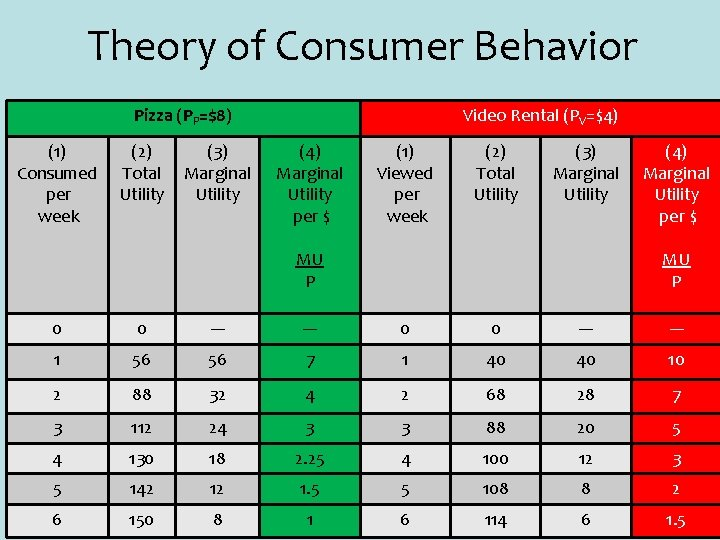Theory of Consumer Behavior Pizza (PP=\$8) (1) Consumed per week (2) Total Utility (3) Marginal Utility Video Rental (PV=\$4) (4) Marginal Utility per \$ (1) Viewed per week (2) Total Utility (3) Marginal Utility MU P (4) Marginal Utility per \$ MU P 0 0 --- --- 1 56 56 7 1 40 40 10 2 88 32 4 2 68 28 7 3 112 24 3 3 88 20 5 4 130 18 2. 25 4 100 12 3 5 142 12 1. 5 5 108 8 2 6 150 8 1 6 114 6 1. 5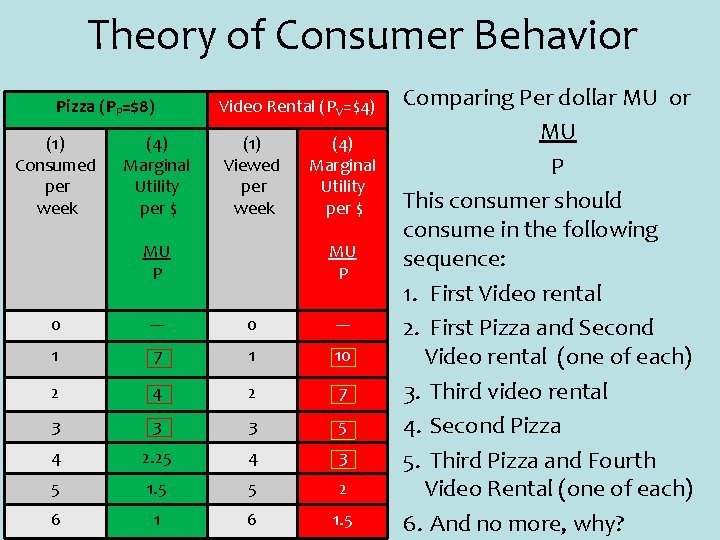Theory of Consumer Behavior Pizza (PP=\$8) (1) Consumed per week (4) Marginal Utility per \$ Video Rental (PV=\$4) (1) Viewed per week MU P (4) Marginal Utility per \$ MU P 0 --- 1 7 1 10 2 4 2 7 3 3 3 5 4 2. 25 4 3 5 1. 5 5 2 6 1. 5 Comparing Per dollar MU or MU P This consumer should consume in the following sequence: 1. First Video rental 2. First Pizza and Second Video rental (one of each) 3. Third video rental 4. Second Pizza 5. Third Pizza and Fourth Video Rental (one of each) 6. And no more, why?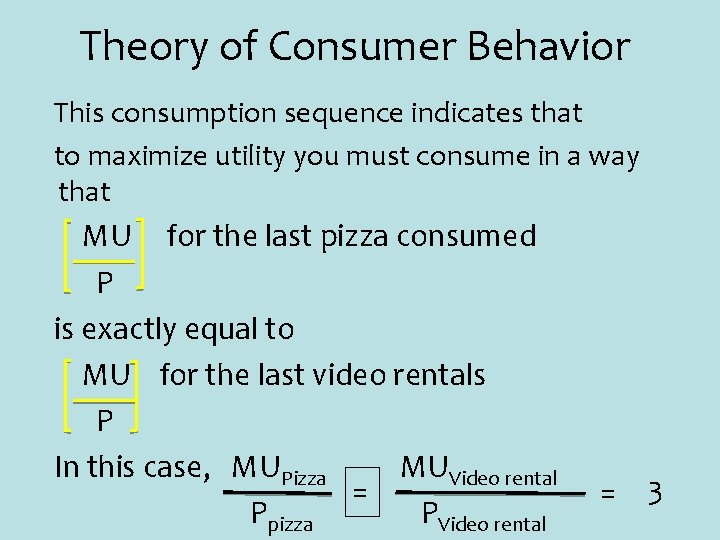Theory of Consumer Behavior This consumption sequence indicates that to maximize utility you must consume in a way that MU for the last pizza consumed P is exactly equal to MU for the last video rentals P In this case, MUPizza MUVideo rental = Ppizza PVideo rental = 3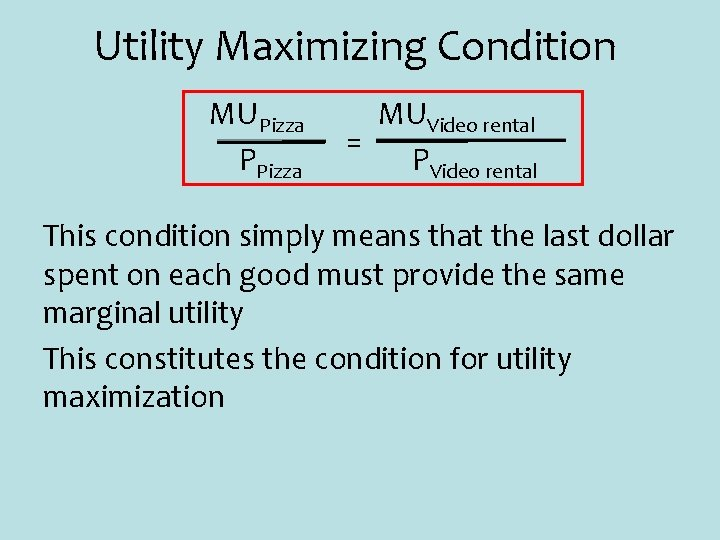Utility Maximizing Condition MUPizza PPizza MUVideo rental = PVideo rental This condition simply means that the last dollar spent on each good must provide the same marginal utility This constitutes the condition for utility maximization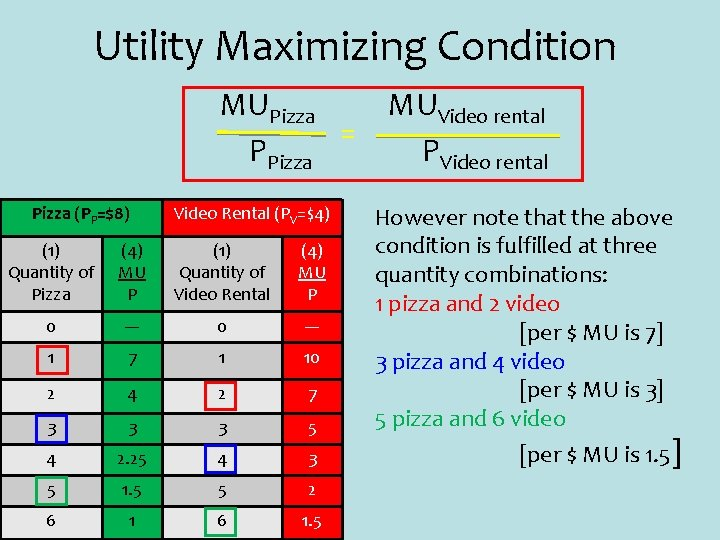Utility Maximizing Condition MUPizza = PPizza (PP=\$8) Video Rental (PV=\$4) (1) Quantity of Pizza (4) MU P (1) Quantity of Video Rental (4) MU P 0 --- 1 7 1 10 2 4 2 7 3 3 3 5 4 2. 25 4 3 5 1. 5 5 2 6 1. 5 MUVideo rental PVideo rental However note that the above condition is fulfilled at three quantity combinations: 1 pizza and 2 video [per \$ MU is 7] 3 pizza and 4 video [per \$ MU is 3] 5 pizza and 6 video [per \$ MU is 1. 5]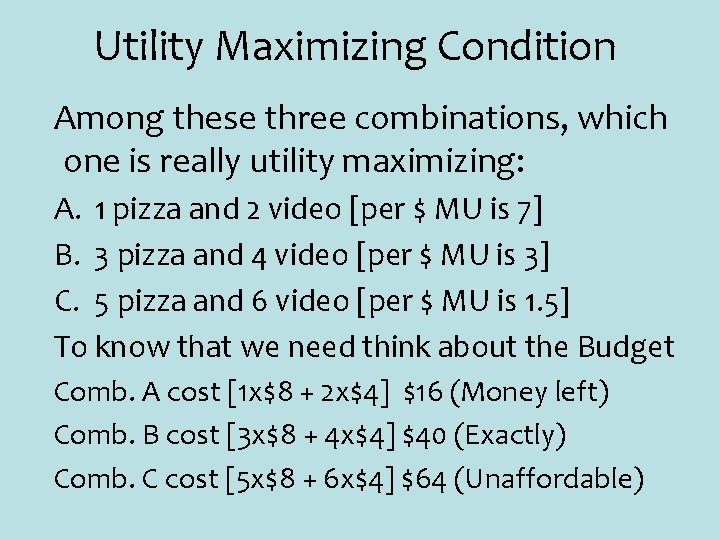Utility Maximizing Condition Among these three combinations, which one is really utility maximizing: A. 1 pizza and 2 video [per \$ MU is 7] B. 3 pizza and 4 video [per \$ MU is 3] C. 5 pizza and 6 video [per \$ MU is 1. 5] To know that we need think about the Budget Comb. A cost [1 x\$8 + 2 x\$4] \$16 (Money left) Comb. B cost [3 x\$8 + 4 x\$4] \$40 (Exactly) Comb. C cost [5 x\$8 + 6 x\$4] \$64 (Unaffordable)Equilibrium Quantity § When the last dollar spent on each good yields the same marginal utility, there is no way to increase utility by reallocating the budget to buy some other quantity combination § This is why quantity choice at which the utility maximized is also called Equilibrium quantityEquilibrium Quantity § How do we check that equilibrium quantity indeed provided highest level of utility possible § To see that we need to go back to the total utility table § Let’s compare another bundle that also costs \$4 o in the utility tableEquilibrium Quantity Pizza (PP=\$8) Video Rental (PV=\$4) (1) Quantity (2) TU (4) MU P 0 0 --- 1 56 7 1 40 10 2 88 4 2 68 7 3 112 3 3 88 5 4 130 2. 25 4 100 3 5 142 1. 5 5 108 2 6 150 1 6 114 1. 5 At the equilibrium combination TU is 212 Equilibrium Quantity suggests that no other affordable quantity choice will yield higher TU Note, 2 pizza and 6 video also cost \$40 But the TU is 202Violation of Utility Max. Condition § The utility maximizing condition is violated when at the current quantity choice one of the following happens: MUPizza PPizza > MUVideo rental PVideo rental < MUVideo rental PVideo rental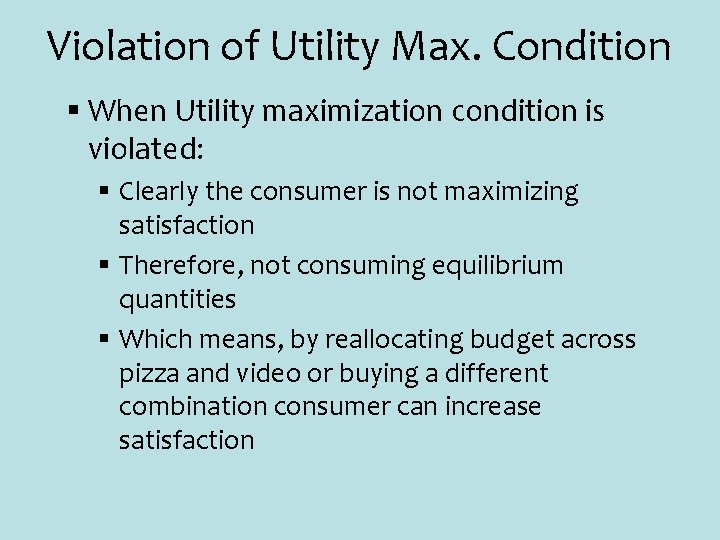Violation of Utility Max. Condition § When Utility maximization condition is violated: § Clearly the consumer is not maximizing satisfaction § Therefore, not consuming equilibrium quantities § Which means, by reallocating budget across pizza and video or buying a different combination consumer can increase satisfactionViolation of Utility Max. Condition § Assume that at the current level of pizza and video consumption, we have MUP MUV > PP PV § What specifically would you suggest this consumer to increase satisfaction?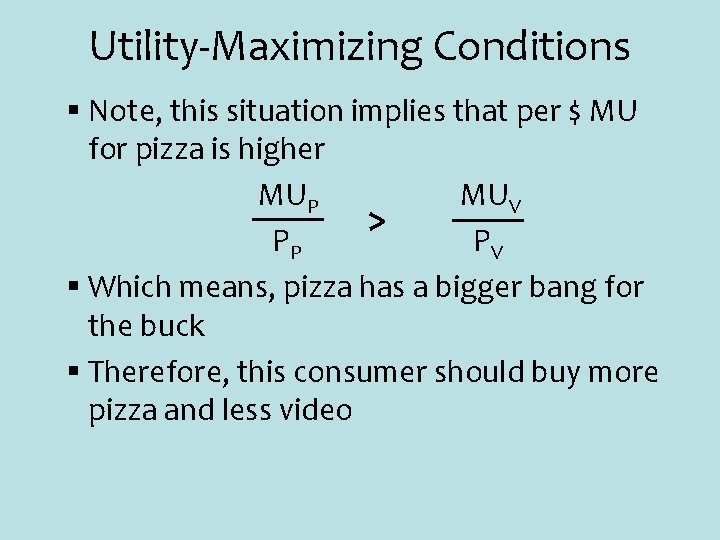Utility-Maximizing Conditions § Note, this situation implies that per \$ MU for pizza is higher MUP MUV > PP PV § Which means, pizza has a bigger bang for the buck § Therefore, this consumer should buy more pizza and less videoUtility-Maximizing Conditions MUP MUV > PP PV § How does buying more pizza and less video restore utility maximizing condition § What happens to MUP when more pizza is consumed? It goes down! § What happens to MUV when less video is consumed? It goes up! § Therefore, by reallocating this way consumer will reach equilibrium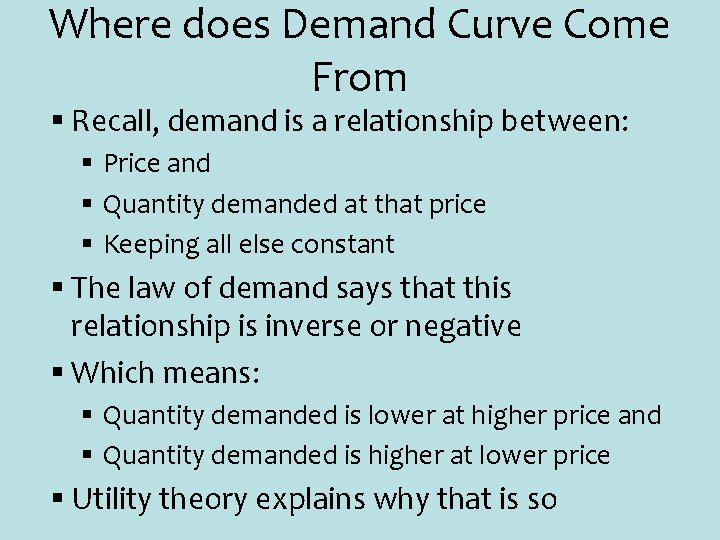Where does Demand Curve Come From § Recall, demand is a relationship between: § Price and § Quantity demanded at that price § Keeping all else constant § The law of demand says that this relationship is inverse or negative § Which means: § Quantity demanded is lower at higher price and § Quantity demanded is higher at lower price § Utility theory explains why that is so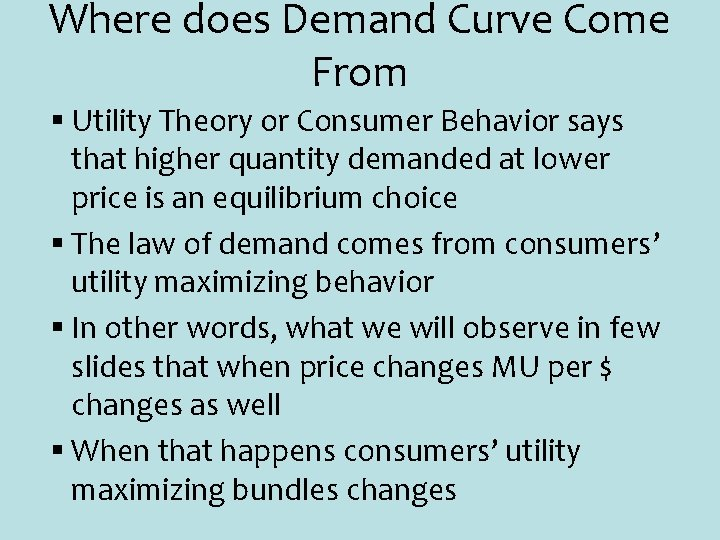Where does Demand Curve Come From § Utility Theory or Consumer Behavior says that higher quantity demanded at lower price is an equilibrium choice § The law of demand comes from consumers’ utility maximizing behavior § In other words, what we will observe in few slides that when price changes MU per \$ changes as well § When that happens consumers’ utility maximizing bundles changes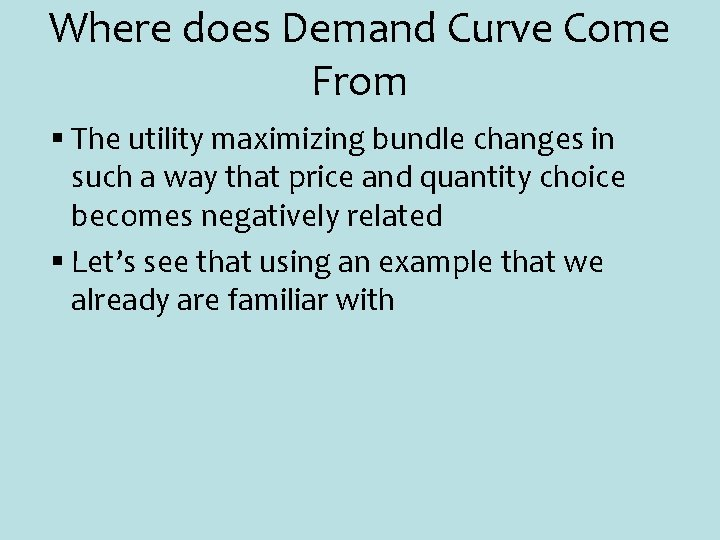Where does Demand Curve Come From § The utility maximizing bundle changes in such a way that price and quantity choice becomes negatively related § Let’s see that using an example that we already are familiar with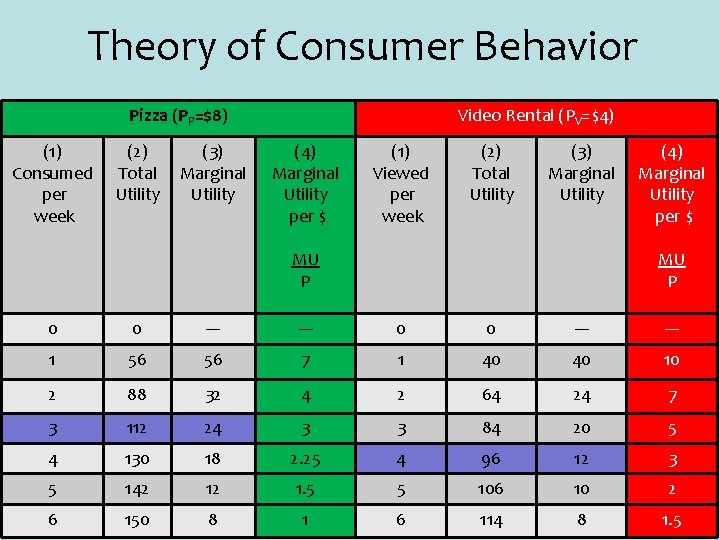Theory of Consumer Behavior Pizza (PP=\$8) (1) Consumed per week (2) Total Utility (3) Marginal Utility Video Rental (PV=\$4) (4) Marginal Utility per \$ (1) Viewed per week (2) Total Utility (3) Marginal Utility MU P (4) Marginal Utility per \$ MU P 0 0 --- --- 1 56 56 7 1 40 40 10 2 88 32 4 2 64 24 7 3 112 24 3 3 84 20 5 4 130 18 2. 25 4 96 12 3 5 142 12 1. 5 5 106 10 2 6 150 8 1 6 114 8 1. 5Where does Demand Curve Come From § Based on this table, from our previous analysis we know that this consumer will choose 3 pizza and 4 video rental § This is because that choice is utility maximizing equilibrium quantity choice § Now, if I want to draw the demand curve for video rental for this consumer, I will have one point of his demand curve § That is PV=4 and Q*V=4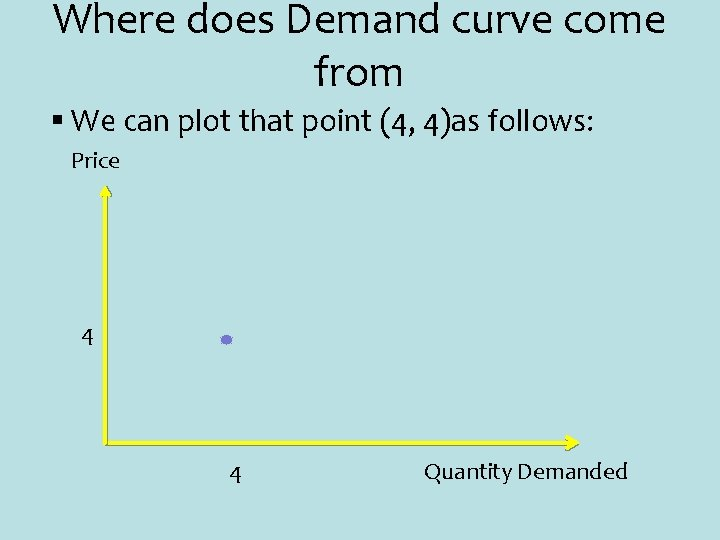Where does Demand curve come from § We can plot that point (4, 4)as follows: Price 4 4 Quantity Demanded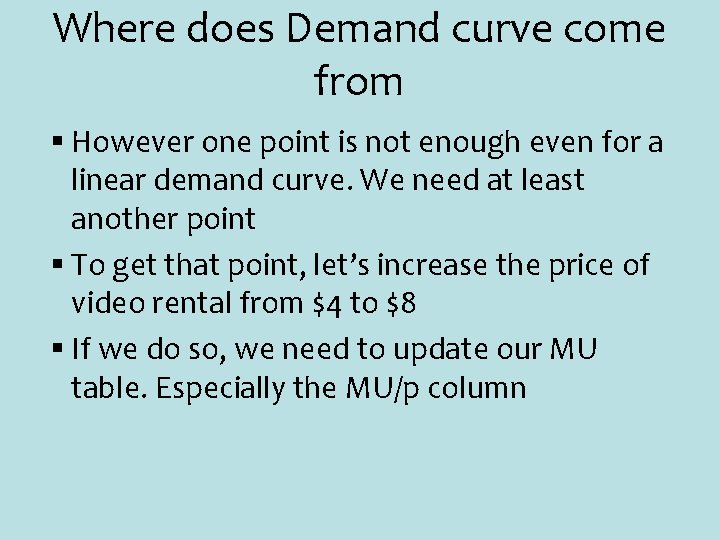Where does Demand curve come from § However one point is not enough even for a linear demand curve. We need at least another point § To get that point, let’s increase the price of video rental from \$4 to \$8 § If we do so, we need to update our MU table. Especially the MU/p columnTheory of Consumer Behavior Pizza (PP=\$8) Video Rental (PV=\$4) (1) (2) (3) (4) (5) Quantity TU MU MU P When P=4 When P=8 0 0 --- --- --- 1 56 56 7 1 40 40 10 5 2 88 32 4 2 64 24 6 3 3 112 24 3 3 84 20 5 2. 5 4 130 18 2. 25 4 96 12 3 1. 5 5 142 12 1. 5 5 108 8 2 1. 25 6 150 8 1 6 114 6 1. 5 1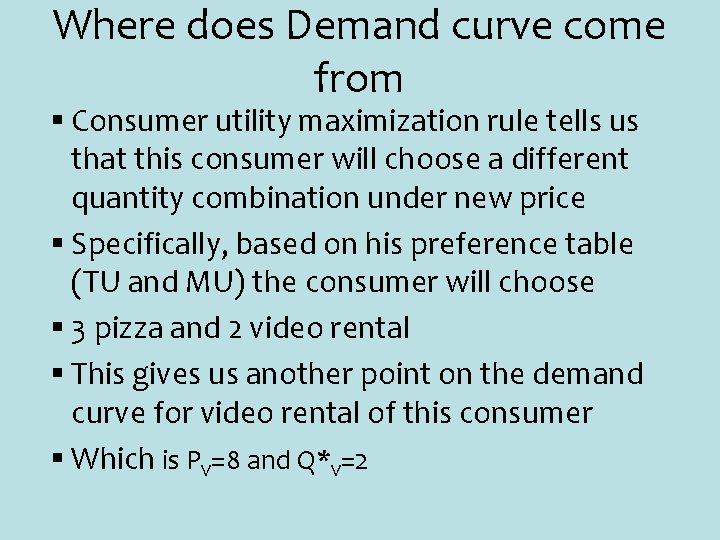Where does Demand curve come from § Consumer utility maximization rule tells us that this consumer will choose a different quantity combination under new price § Specifically, based on his preference table (TU and MU) the consumer will choose § 3 pizza and 2 video rental § This gives us another point on the demand curve for video rental of this consumer § Which is PV=8 and Q*V=2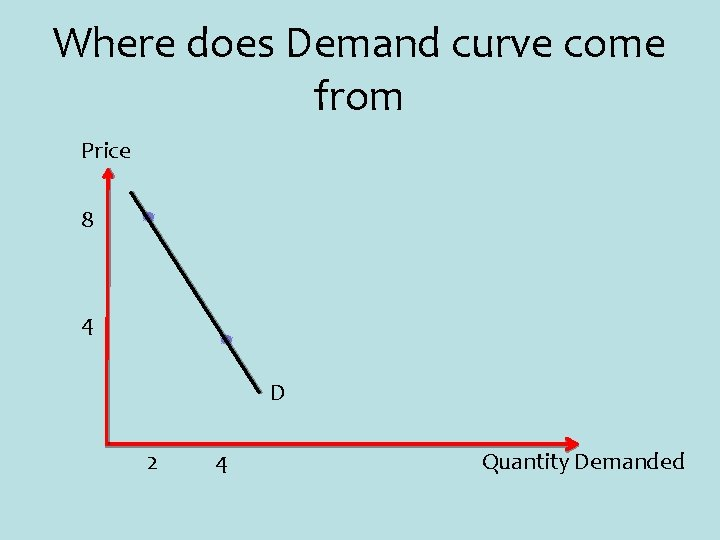Where does Demand curve come from Price 8 4 D 2 4 Quantity Demanded